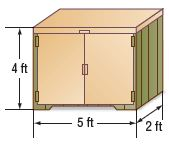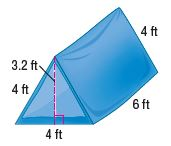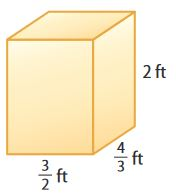Homework Explained - Math Practice 101Dear guest, you are not a registered member. As a guest, you only have read-only access to our books, tests and other practice materials.

As a registered member you can:

Registration is free and doesn't require any type of payment information. Click here to Register.
Go to page:
Chapter 10:Area; Mid-Chapter Review

### Problem Solving

• Question 1

An office building is built in the shape of a rectangular prism. It has a length of 168 yards, a width of 115 yards, and a height 96 yards. What is the volume of the building?

•  V = $$\text{ yd}^3$$
• Question 2

Liza is researching packaging options for her shop. She wants to know the volume of a triangular prism 9 inches tall with a triangular base that is 3 inches by 2 inches.

•  V = $$\text{ in}^3$$
• Question 3

Use Math Tools Justin is building a storage trunk like the one shown. How much wood is needed to make the trunk?•  $$\text{ ft}^2$$
• Question 4

A baker needs to put icing on a rectangular cake. The cake is 14 inches long, 12 inches wide, and 4 inches tall. What is the surface area of the cake, not including the bottom?

•  The surface area of the cake = $$\text{ in}^2$$
• Question 5

Stephanie was making a tent like the one shown. How much fabric does she need to make the tent?•  $$\text{ ft}^2$$
• Question 6

Reason Abstractly A paper die has sides that are all equilateral triangles. Each triangle has a side length of 1.5 cm. The slant height is 1.3 cm. Find the surface area of the die.

•  $$\text{ cm}^2$$

### Moving Time

The Davidsons are moving into a new house and have rented a trailer to transport boxes for the move. They bought cardboard boxes, like the one shown, in which they will pack their belongings. The trailer has 180 cubic feet of cargo space. The height of the trailer is 7.5 feet and the width is 4 feet.The perimeter of the base of the trailer is 20 feet.• Question 7

Part A

The Davidsons need to know the dimensions of the trailer so they can maximize space while packing boxes. What is the length and the width of the trailer in feet?

•  w = l =
• Question 7

Part B

If the boxes can be put in the trailer in any position, what is the greatest number of boxes that will fit in the trailer? How many boxes will fit in the trailer if each box must be loaded as shown in the image (with 2 feet as the height).

•  boxes
• Question 7

Part C

The family is taking three gift-wrapped presents (using the same boxes). How much wrapping paper will they need? Draw a net to represent one of the boxes.

•  $$\text{ ft}^2$$

Yes, email page to my online tutor. (if you didn't add a tutor yet, you can add one here)